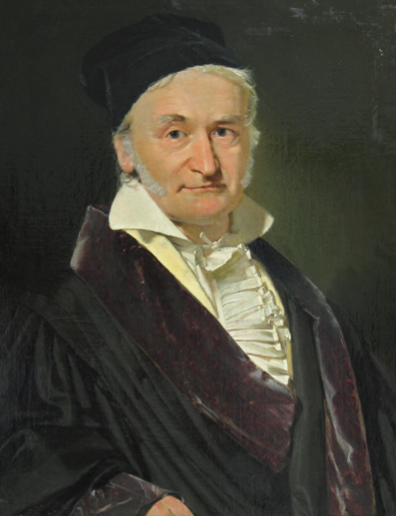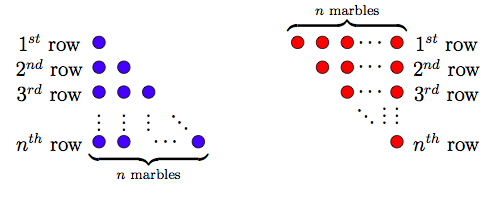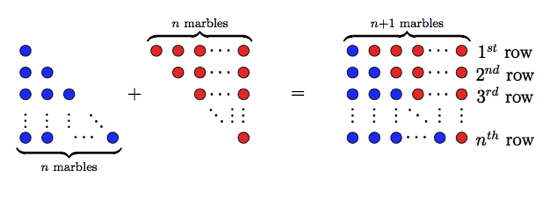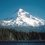# This note has been used to help create the Gauss: The Prince of Mathematics wikiAs you progress further into college math and physics, no matter where you turn, you will repeatedly run into the name Gauss. Johann Carl Friedrich Gauss is one of the most influential mathematicians in history. Gauss was born on April 30th, 1777 in a small German city north of the Harz mountains named Braunschweig. The son of peasant parents (both were illiterate), he developed a staggering number of important ideas and had many more named after him. Many have referred to him as the princeps mathematicorum, or the “Prince of Mathematics.”

As part of his doctoral dissertation (at the age of 21), Gauss was one of the first to prove the fundamental theorem of algebra. He went on to publish seminal works in many fields of mathematics including number theory, algebra, statistics, analysis, differential geometry, geodesy, geophysics, electrostatics, astronomy, optics, etc. Number theory was Gauss’s favorite and he referred to number theory as the “Queen of Mathematics”.

One of the reasons why Gauss was able to contribute so much math over his lifetime was that he got a very early start. There are many tales of his childhood precociousness. The most famous anecdote of young Gauss, is the time he found the shortcut for calculating sums of an arithmetic progression at the tender age of 10.

One day at school, Gauss's teacher wanted to take a rest and asked the students to sum the integers from 1 to 100 as busy work. After a few seconds, the teacher saw Gauss sitting idle. When asked why he was not frantically doing addition, Gauss quickly replied that the sum was 5050. His classmates and teacher were astonished, and Gauss ended up being the only pupil to calculate the correct answer.

$1+2+3+ \cdots +(n-1) = ?$

The story may be apocryphal, and is told different ways in different sources. Nobody is sure which method of summing an arithmetic sequence Gauss figured out as a child. Though there are several ways young Gauss might have solved it, one of them has a concise, intuitive, and elegant visual representation.Arithmetic Progression

Consider two sets of marbles as shown in the Figure 1. The left pile has $n$ rows of blue marbles, where the $j$th row contains $j$ marbles. The right pile has $n$ rows of red marbles, where the $j$th row contains $n+1-j$ marbles.

The total number of blue marbles is given by

$1+2 +3+ \cdots + (n-1)+n,$

while the total number of red marbles is given by

$n+(n-1)+(n-2) + \cdots + 2 + 1,$

and clearly both contain the same number of marbles. Now if we were to add these piles together as shown in Figure 2, we would then get a stack with $n$ rows, where each row contains $n + 1$ marbles.Arithmetic Progression

The total number of marbles in the added pile would be $n(n + 1)$. Since both the red pile and the blue pile have an equal number of marbles, each pile must have contributed $\frac{n(n + 1)}{2}$ marbles. Hence, we obtain:

$1+2+3+...+(n-1)+n= \frac{n(n-1)}{2}.$

To sum all the numbers from 1 to 100, Gauss simply calculated $\frac{100\times (100+1)}{2}=5050$, which is immensely easier than adding all the numbers from 1 to 100. All sane humans would rather do one addition, one multiplication, and one division than do lots of tedious addition operations. Note that $1+2+3 + \ldots +(n-1)+n$ must always be a natural number. Even though the above formula divides by 2, the result will always be a natural number. This is because the numerator will always be conveniently even due to the multiplication properties of parity. For example, $n$ could either be even or odd. If $n$ is even, then $n+1$ is odd and hence

$n \times (n + 1) = even \times odd = even.$

Similarly, if $n$ is odd, then $n + 1$ is even and hence

$n \times (n + 1) = odd \times even = even.$

Therefore, the numerator is always even and $n(n + 1)$ is always a natural number.

Numbers of the form $\frac{n(n + 1)}{2}$ are called triangular numbers, for reasons well illustrated in the above figures. The first few triangular numbers are

$1,3,6,10,15,21,28,36, \ldots.$

It is commonplace to encounter an application of summing an arithmetic sequence, both in classroom problems, and in describing the broader world. It is less common to meet 10 year olds who figure out the tricks of arithmetic progression for themselves. It is even less common for a precocious 10 year old, to grow up to be nearly as prolific as Gauss. Check out this list of things named after Gauss.Note by Peter Taylor
7 years, 4 months ago

This discussion board is a place to discuss our Daily Challenges and the math and science related to those challenges. Explanations are more than just a solution — they should explain the steps and thinking strategies that you used to obtain the solution. Comments should further the discussion of math and science.

When posting on Brilliant:

• Use the emojis to react to an explanation, whether you're congratulating a job well done , or just really confused .
• Ask specific questions about the challenge or the steps in somebody's explanation. Well-posed questions can add a lot to the discussion, but posting "I don't understand!" doesn't help anyone.
• Try to contribute something new to the discussion, whether it is an extension, generalization or other idea related to the challenge.

MarkdownAppears as
*italics* or _italics_ italics
**bold** or __bold__ bold
- bulleted- list
• bulleted
• list
1. numbered2. list
1. numbered
2. list
Note: you must add a full line of space before and after lists for them to show up correctly
paragraph 1paragraph 2

paragraph 1

paragraph 2

[example link](https://brilliant.org)example link
> This is a quote
This is a quote
    # I indented these lines
# 4 spaces, and now they show
# up as a code block.

print "hello world"
# I indented these lines
# 4 spaces, and now they show
# up as a code block.

print "hello world"
MathAppears as
Remember to wrap math in $$ ... $$ or $ ... $ to ensure proper formatting.
2 \times 3 $2 \times 3$
2^{34} $2^{34}$
a_{i-1} $a_{i-1}$
\frac{2}{3} $\frac{2}{3}$
\sqrt{2} $\sqrt{2}$
\sum_{i=1}^3 $\sum_{i=1}^3$
\sin \theta $\sin \theta$
\boxed{123} $\boxed{123}$

Sort by:

And then there's the story about the mathematician John von Neumann, asked to find out how far a fly flew back and forth between two trains approaching each other before they collided. He struggled for a few seconds mentally before he came up with the correct answer. "Interesting,", the person who posed the problem said, "Most people try to sum the infinite series". John von Neumann is said to have replied, "What do you mean? That's how I did it!"

- 6 years, 8 months ago

John von Neumann discovered what ?

- 6 years, 8 months ago

John von Neumann was one of the top mathematicians in the 20th century. He's most famous for having put quantum mechanics on a rigorous mathematical foundation in the early days when physicists were fumbling around trying to make sense out of it. In his lifetime, like Gauss, he's made a long list of contributions to mathematics, logic, physics, economics and game theory, and was one of the physicists that was involved in the development of nuclear weapons.

- 6 years, 8 months ago

Not to mention his contributions to computer science.

- 5 years, 12 months ago

True, that. There's hardly anything that he did not touch. Herman Kahn, which Dr Strangelove is supposed to be based on, is said to have coined the term "Mutual Assured Destruction", but it was actually John von Neuman who first coined it, as a matter of game theory.

- 5 years, 12 months ago

Euler is king of mathematics!!!!!!! e^k

- 6 years, 8 months ago

No wonder he formulated the Gauss's theorem!

- 5 years, 12 months ago